• C++实现函数atoi() ...// 保存字符串转换为数字的结果 bool isNegative = 0;// 判断字符串中是否存在正负号 int n = 0; const char* p = str; if (p == nullptr) return -1;// 字符串空则转为

用C++实现函数atoi()

#include<iostream>
#include<cmath>
using namespace std;

int myAtoi(const char* str) {
int num = 0;// 保存字符串转换为数字的结果
bool isNegative = 0;// 判断字符串中是否存在正负号
int n = 0;
const char* p = str;
if (p == nullptr) return -1;// 字符串为空则转为-1
while (*p++ != '\0') {
n++;
}
p = str;
if (p == '-') {
isNegative = 1;// 首字母是负号
}
char m = '0';
for (int i = 0; i < n; i++) {
char m = *p++;
if (m < '0' || m > '9') {
continue;// 遇到非数字
}
if (num != 0 || m != '0') {// 过滤字符串开始的0字符
m -= 0x30;// 将数字字符转换为数值
num += m *int(pow(10, n - 1 - i));
}
}
if (isNegative) return (0 - num);// 如果字符串中有负号，则将转换的数值取反
else return num;// 字符串没有负号即正数得到真正的数值
}

int main()
{
char* s;
cout << "请输入要转换的字符串: ";
cin >> s;
int result = myAtoi(s);
cout << "转换后的数字为: " << result << endl;
return 0;
}

测试结果: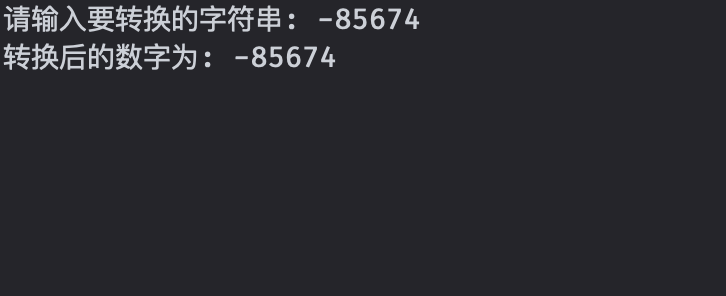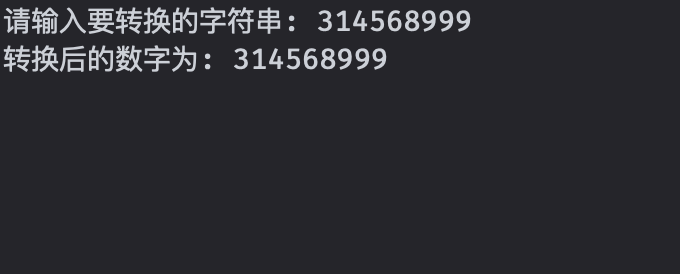展开全文c++
• 2.使用stoi()或atoi()进行字符串转换 二：将数字转化字符串 1.数字转化字符串：加 ‘0’ ，然后逆序。 2.数字转字符串 sprintf(str, “%d”, num); 三：将数字转化成字符 1.int转char 类型 和数字字符转数字相反...

一：将字符串转化成数字
1.使用字符串流stringstream来做类型转化。stingstream能将任何类型轻松转变为字符串类型，也能将字符串类型转变为数字类型
2.使用stoi()或atoi()进行字符串转换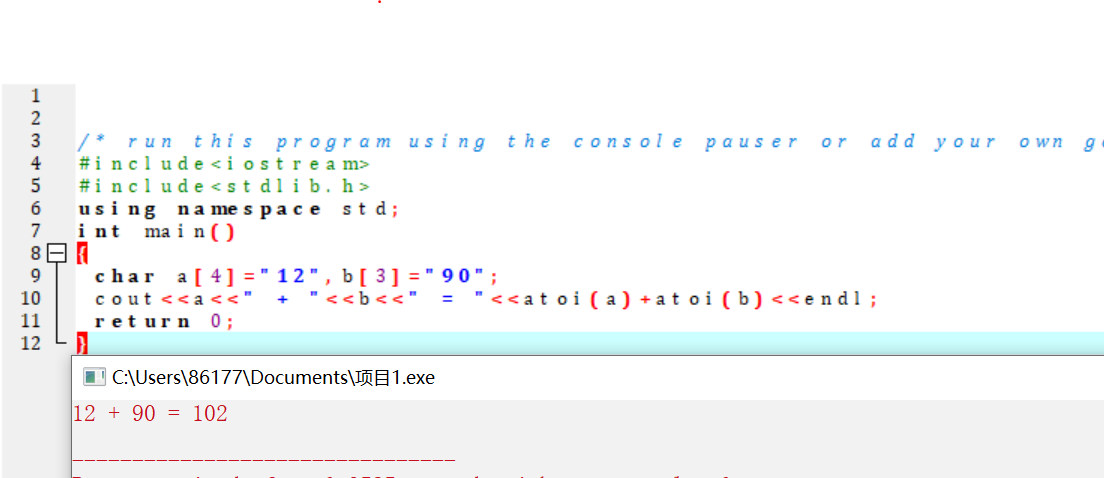二：将数字转化字符串
1.数字转化为字符串：加 ‘0’ ，然后逆序。
2.数字转字符串
sprintf(str, “%d”, num);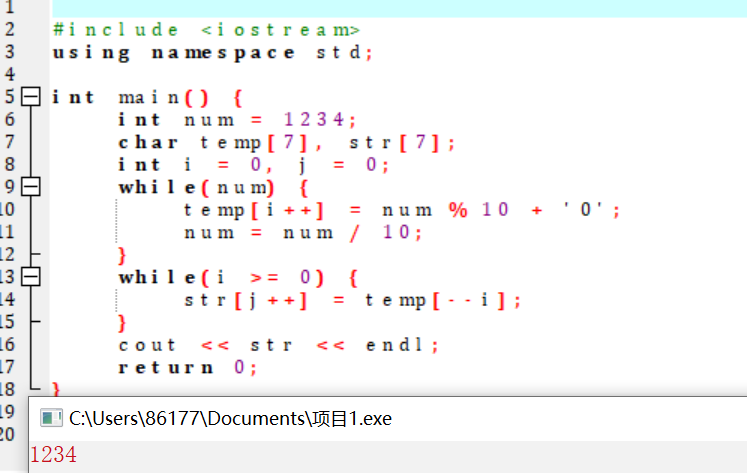三：将数字转化成字符
1.int转char 类型 和数字字符转数字相反 我们可以加上 ‘0’的ASCII 就可以变为char类型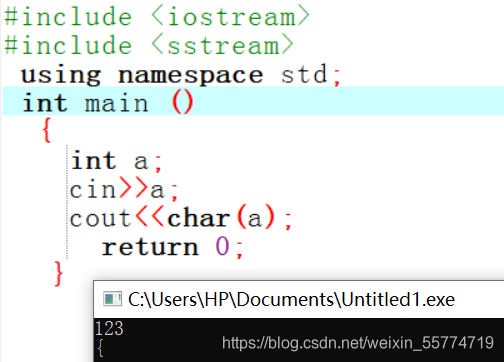四：将字符转化成数字字符
1.减 ‘0’隐性转化为int类型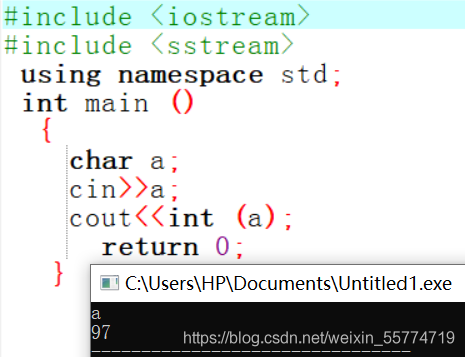展开全文字符串 c++
• C++将字符串变为数字

千次阅读 2021-03-20 10:25:45
将字符串变为数字 简单粗暴法 如果是"123"这种的，大概只要直接利用数字的ASII码来相减就可以了 string str = "123"; int num = 0; for(auto &s：str){ num = num*10 + s-'0'; } 遇到带有符号的 但是"-100...

将字符串变为数字

简单粗暴法

如果是"123"这种的，大概只要直接利用数字的ASII码来相减就可以了

string str = "123";
int num = 0;
for(auto &s：str){
num  = num*10 + s-'0';
}

遇到带有符号的

但是"-100"这种，怎么直接换为数字？

用atoi来将字符数组转换为数字，atoi这个函数可以pass掉那些空格" ",并且可以加号和负号一同变为数字。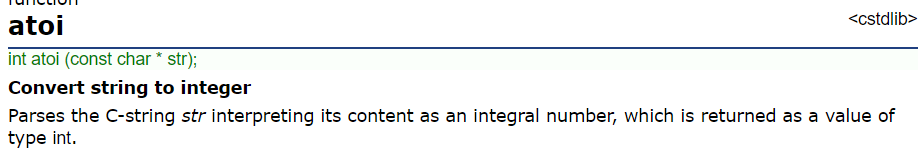string str = "-100";
int num = atoi(str); // 这样是不行的，因为atoi不支持直接把c++的字符串改为数字，只能先变为字符数组才行

正确做法，通过c_str将string变为字符数组，再用atoi来变为数字

string str = "-100";
int num = atoi(str.c_str());

展开全文• 将字符串转换为整型 #include<iostream> #include<string> using namespace std; int main() { int n; string s1="89"; n=stoi(s1); cout<<n<<endl; return 0; } 二、stoll() ...

一、stoi()

将字符串转换为整型

#include<iostream>
#include<string>
using namespace std;

int main()
{
int n;
string s1="89";
n=stoi(s1);
cout<<n<<endl;
return 0;
}

二、stoll()

将字符串转换为long long

#include<iostream>
#include<string>
using namespace std;

int main()
{
long long n;
string s1="874959387578949";
n=stoll(s1);
cout<<n<<endl;
return 0;
}

三、stof()

将字符串转换为float型

#include<iostream>
#include<string>
using namespace std;

int main()
{
float n;
string s1="3.1678";
n=stof(s1);
cout<<n<<endl;
return 0;
}

四、stod()

将字符串转换为double型

#include<iostream>
#include<string>
using namespace std;

int main()
{
double n;
string s1="3.1678";
n=stod(s1);
cout<<n<<endl;
return 0;
}

展开全文字符串
• C语言atoi()函数：将字符串转换成int(整数)头文件：#include atoi() 函数用来将字符串转换成整数(int)，其原型：int atoi (const char * str);【函数说明】atoi() 函数会扫描参数 str 字符串，跳过前面的空白字符...
• 能完成字符串数字转换的方法...字符串数字的各个函数还可以选择从字符串的哪个位置开始转换转换成整型量还可以选择不同的进制，具体见C++primer 328页。 2.使用stringstream 首先要包含sstream头文件，这个类继承c++ 字符串 算法
• #include <iostream> #include <algorithm> //必需 using namespace std; int main() ... transform(str.begin(),str.end(),str.begin(),::...//将字符串全部转换为大写字母 // ::tolower转换为小写字母c++ c语言
• C 库函数 int atoi(const char *str) 把参数 str 所指向的字符串转换为一个整数（类型 int 型）。 声明 下面是 atoi() 函数的声明。 int atoi(const char *str) 参数 str – 要转换整数的字符串。 返回值 该...c++
• 字符串转化成整数 题目描述： 思路： 要考虑正负数 要考虑数据是否溢出 题目来源：牛客 class Solution { public: int StrToInt(string str) { int len=str.size(); int flag=1; if(len==0) ...字符串 c++
• 把一个字符串转成整数，这看起来是道很简单的题目，实现其基本功能大部分人能用10行之内的代码来实现。可是，当我们把很多特殊情况，即测试用例都考虑进去，这不算一件容易的事情。把空指针null， 空字符串"", 正负...c语言 c++ 开发语言
• 通常，或更具体地说，在竞争性编程中，有许多情况需要将数字转换字符串或将字符串转换为数字。但是缺乏某些必不可少的工具的知识使我们不得不这样做。本文介绍了一些实现此任务的方法。 将字符串转换为数字 方法...c++ c语言 字符串 字符串处理 字符串转换
• 字符串：“1234” 数组：{1234} string s=“1234”; int a={0} int num=0; for(int i=0; i<s.size() ;i++){ //把单个字符变为数字 a[i] = s[i]-‘0’; }c++ c语言
• C++字符串转换

2021-06-08 15:56:57
(1)将字符转换为int，可以使用atoi、_atoi64或atol。 (2)int转换为CString变量，可以使用CString的Format函数。如 CString s; int i = 64; s.Format(”%d”, i) 1.2、CString互转char* (1)char * 转换为CString ...c++ 字符串
• C++数字与字符（串）转换

千次阅读 2021-07-18 14:43:45
C++数字与字符（串）转换一、数字与字符互转1、数字转字符2、字符转数字二、数字字符串互转1、数字字符串2、字符串数字 一、数字与字符互转 1、数字转字符 #include <iostream> using namespace std; ...c++
• 数字字符串，int float类型 同理字符串数字,int float类型 同理二、使用sprintf()函数char str;int a=1234321;sprintf(str,”%d”,a);char str;double a=123.321;sprintf(str,”%.3lf”,a);char str[1.....
• C语言中一些将字符串转换为数字的函数小结C语言atoi()函数：将字符串转换成int(整数)头文件：#include atoi() 函数用来将字符串转换成整数(int)，其原型：int atoi (const char * str);【函数说明】atoi() 函数会...
• 1.都是c++的字符处理函数，把数字字符串转换成int输出 不同点： 1.头文件库不同：stoi()的头文件是<string>;而atoi()的头文件是<stdlib.h> 2.atoi()的参数是const char*,因此对于一个字符串str我们...c++ 字符串
• 代码实现十六进制字符串转换为十进制整数的方法，具体实现如下： /* * 十六进制数字组成的字符串（包含可选的前缀0x或0X）转换与之等价的整型值 */ #include <stdio.h> #include <math.h> /*...C语言 c++ 字符串 整数 转换
• c++将数字变成字符串的方法

千次阅读 2020-12-30 10:32:39
c++将数字变成字符串的方法 方法一： int num=11; string num1; stringstream ss; ss<<num; ss>>num1; 方法二： int num=11; cout<<to_string(num)<<endl;字符串
• 就是遍历判断这个字符串，每个字符十分符合ASCii数字的范围。 摘抄如下： bool AllisNum(string str) { for (int i = 0; i < str.size(); i++) { int tmp = (int)str[i]; if (tmp >= 48 && ...c语言 c++
• ecvt()：双精度浮点型值转换为字符串转换结果中不包含十进制小数点。 fcvt()：指定位数为转换精度，其余同ecvt()。 这里只进行演示了itoa 和 gcvt。 # include int main () { int num_int = 435; double num_...字符串 c++
• 本文介绍二进制、十进制、十六进制及字符串的相互转换方法。 代码 #include <bits/stdc++.h> using namespace std; int main() { int decNum = 255; /* 10进制转2进制 */ bitset<32> a(decNum); ...
• C++将数字转化成字符串

千次阅读 2021-02-27 11:07:02
#include <iostream> #include <sstream> using namespace std; int main() { ostringstream os; int i = 1234; os << i; std::string str = os.str(); cout <<...}
• C++编程中，时常会遇到数值和字符串之间的转换，C-Style的atoi，atol，strtod，strtol，strtoul等函数在功能上有时候会略显不足，当然在C++中实现数值和字符串转换的形式各种各样，本文对自己在编程中遇到的情况...
• 1、字符串数字之间的转换(1)string --> char *string str("OK");char * p = str.c_str();(2)char * -->stringchar *p = "OK";string str(p);(3)char * -->CStringchar *p ="OK";CString m_Str(p);//或者...
• 一、数字字符串 头文件 #include<string> #include<typeinfo> 1.1 int型数字字符串 int num = 123; string num2str = to_string(num); cout << typeid(to_string(num) == typeid(string) <...
• 项目中上位机给下位机发送的指令是...//HexChar()16进制字符转换为相应大小的16进制数 char HexChar(char c) { if((c>='0')&&(c<='9')) return c-'0'; else if((c>='A')&&(c<='F'))c++
• c++ 字符串转换为数字 利用的是标准库中的 <stdlib.h> ，下面测试内容： #include <iostream> #include <stdlib.h> //要包含的头文件 using namespace std; char* test(); int main(int argc...c++ 字符串
• 最近正好学了文件相关的操作，顺便记录一下。...题目大致意思就是把一个文件里的数字字符通过转化并加一写到另一个文件里，遇到不全是数字字符的字符串则不用处理。 代码如下： 新的改变 我们对Markdown编c++ 字符串
• 在用过C#一段时间后，发现C#里面string+数值的方法特别方便，但是在C++里面却没有内置这样的运算。本文接下来会介绍如何通过重载运算符+来实现上述功能。c++ 字符串...

c++将字符串转换为数字c++ 订阅